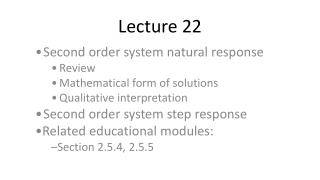DownloadDownload PresentationLecture 22

# Lecture 22

Télécharger la présentation## Lecture 22

- - - - - - - - - - - - - - - - - - - - - - - - - - - E N D - - - - - - - - - - - - - - - - - - - - - - - - - - -
##### Presentation Transcript

1. Lecture 22 Second order system natural response Review Mathematical form of solutions Qualitative interpretation Second order system step response Related educational modules: Section 2.5.4, 2.5.5

2. Second order input-output equations • Governing equation for a second order unforced system: • Where •  is the damping ratio (  0) • n is the natural frequency (n  0)

3. Homogeneous solution – continued • Solution is of the form: • With two initial conditions: ,

4. Damping ratio and natural frequency • System is often classified by its damping ratio, : •  > 1  System is overdamped (the response has two time constants, may decay slowly if  is large) •  = 1  System is critically damped (the response has a single time constant; decays “faster” than any overdamped response) •  < 1  System is underdamped (the response oscillates) • Underdamped system responses oscillate

5. Overdamped system natural response • >1: • We are more interested in qualitative behavior than mathematical expression

6. Overdamped system – qualitative response • The response contains two decaying exponentials with different time constants • For high , the response decays very slowly • As  increases, the response dies out more rapidly

7. Critically damped system natural response • =1: • System has only a single time constant • Response dies out more rapidly than any over-damped system

8. Underdamped system natural response • <1: • Note: solution contains sinusoids with frequency d

9. Underdamped system – qualitative response • The response contains exponentially decaying sinusoids • Decreasing  increases the amount of overshoot in the solution

10. Example • For the circuit shown, find: • The equation governing vc(t) • n, d, and  if L=1H, R=200, and C=1F • Whether the system is under, over, or critically damped • R to make  = 1 • Initial conditions if vc(0-)=1V and iL(0-)=0.01A

11. Part 1: find the equation governing vc(t)

12. Part 3: Is the system under-, over-, or critically damped? • In part 2, we found that  = 0.2

13. Part 4: Find R to make the system critically damped

14. Part 5: Initial conditions if vc(0-)=1V and iL(0-)=0.01A

15. Simulated Response